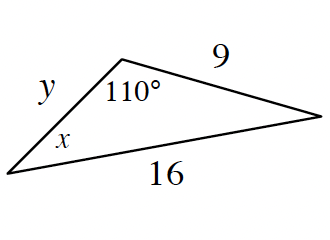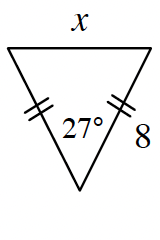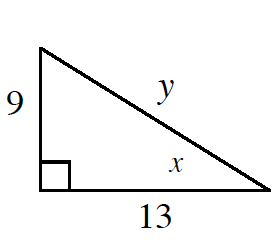### Home > GC > Chapter 10 > Lesson 10.1.4 > Problem10-39

10-39.

For each triangle below, solve for the given variables.

1.What law can you use to solve for the two variables?

$\frac{\text{sin}x}{9}=\frac{\text{sin}110}{16}$

$16(\sin x) = (\sin 110)9$

$x ≈ 31.9º$
$y ≈ 10.5 \; \text{in.}$

1.Use the Law of Cosines.

$x ≈ 3.7 \; \text{m}$

1.What trig ratio can you use to find $x$? Use the Pythagorean Theorem to find $y$.

$x ≈ 34.7º$
$y = \sqrt{250} \approx 15.8 \; \text{ft}$